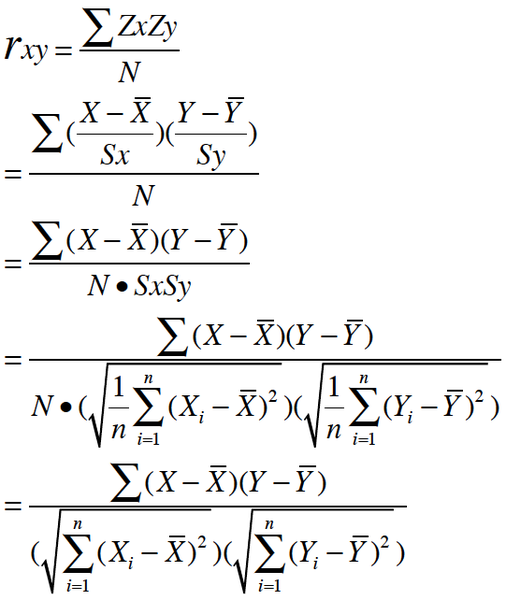# 皮尔逊相关系数的理解

Z分数一般代表正态分布中数据偏离中心点的距离.等于变量减掉平均数再除以标准差.(就是高考的标准分类似的处理)### 皮尔逊相关的约束条件

• 1 两个变量间有线性关系
• 2 变量是连续变量
• 3 变量均符合正态分布,且二元分布也符合正态分布
• 4 两变量独立

### 先举个手算的例子

x<-c(1,2,3,5,8)
y<-c(0.11,0.12,0.13,0.15,0.18)

x的平均数是:3.8
y的平均数是0.138

(1-3.8)*(0.11-0.138)=0.0784
(2-3.8)*(0.12-0.138)=0.0324
(3-3.8)*(0.13-0.138)=0.0064
(5-3.8)*(0.15-0.138)=0.0144
(8-3.8)*(0.18-0.138)=0.1764

0.0784+0.0324+0.0064+0.0144+0.1764=0.308

sum((x-mean(x))^2)=30.8
sum((y-mean(y))^2)= 0.00308

(1-3.8)^2=7.84 #平方
(2-3.8)^2=3.24 #平方
(3-3.8)^2=0.64 #平方
(5-3.8)^2=1.44 #平方
(8-3.8)^2=17.64 #平方

7.84+3.24+0.64+1.44+17.64=30.8

sum((y-mean(y))^2)= 0.00308

30.8^0.5=5.549775
0.00308^0.5=0.05549775

0.308/(5.549775*0.05549775)=1

> x<-rnorm(n=100,mean=35,sd=5)  #创建一组平均数为35,标准差为5,样本数为100的随机数
> y<-rnorm(n=100,mean=45,sd=8) #创建一组平均数为45,标准差为8,样本数为100的随机数
>  cor.test(x,y,method="pearson") #计算这两组数的相关,并进行T检验

Pearson's product-moment correlation

data:  x and y
t = -0.0269, df = 98, p-value = 0.9786
alternative hypothesis: true correlation is not equal to 0
95 percent confidence interval:
-0.1990316  0.1938019
sample estimates:
cor
-0.002719791

cor(x,y)

https://github.com/alexgutteridge/rsr...

https://github.com/davidrichards/stat...

https://github.com/jtprince/simpler

• 0.8-1.0 极强相关
• 0.6-0.8 强相关
• 0.4-0.6 中等程度相关
• 0.2-0.4 弱相关
• 0.0-0.2 极弱相关或无相关08-27
10-218854
07-30658
03-131万+
03-124万+
01-174544
08-281万+
10-135104
09-11697